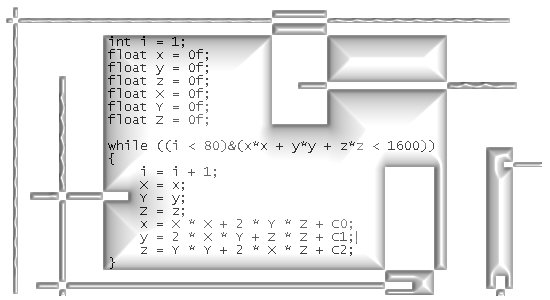# Short stuff on the 3D Mandelbrot fractal.

About a year ago I decided to take about two years to make it to the 3D Mandelbrot set. So I tried to learn one of those modern programming languages like C++, I did build a new computer because on my old system C++ would not run. And so on and so on.

Decades ago I tried to learn a computer programing language known as Basic. When I found out how those kind of programming languages evaluted an integral, I almost had to vomit.
In those long lost years I already developed a fundamental dislike against programming.

Now I am 52 years of age and it is still the same; me writing computer code is not a happy thing to do. So I killed the project of being the first person on this planet to view the 3D Mandelbrot set using the 3D complex or circular multiplication…

I never made it beyond what is in C++ a ConsoleApllication; you get your output in an old fashioned DOS screen and no graphics at all. And how to embed this into a thing you can actually fly through, I have given up on that.

So I did not write much code, but the results had all you expected it should have: Strong sensitivity to initial conditions and so on and so on.

Well here is the kernel of the 3D Mandelbrot set for the circular multiplication.
Circular simply means we are using 3D circular numbers X = x + yj + zj^2 where j^3 = 1.

In this kernel we have to use so called ‘dummy variables’ because computers are so stupid you cannot tell them how to calculate the next round of variables This despite in the year 2016 most desktops have multiple cores, your programming language still uses the old von Neuman principles.

Here is the kernel with the dummy variables written as capital X, Y and Z while we only want to know how the x, y and z evolve over the iterations… :

int i = 1;
float x = 0f;
float y = 0f;
float z = 0f;
float X = 0f;
float Y = 0f;
float Z = 0f;

while ((i < 80)&(x*x + y*y + z*z < 1600))
{
i = i + 1;
X = x;
Y = y;
Z = z;
x = X * X + 2 * Y * Z + C0;
y = 2 * X * Y + Z * Z + C1;
z = Y * Y + 2 * X * Z + C2;
}

__________Oh oh my dear Mandelbrot baby, now I have thrown you into the river I will never be the first human to observe your intrinsic details. Let it be, let it be because for the rest of my life I can still hate that stupid computer code writing.